# Plotting with exuber

### The plotting API has changed in exuber 0.4.0

The new design offers full flexibility and customization to produce publication-ready plots. exuber used to plot graph individually in a list, and then you could modify each plot and arrange them into a single grob with ggarrange()(which now is defunct). However, newer versions of exuber focus on providing a faceted plot as it easier to change the aesthetics and themes.

Let’s start by simulating some data.

set.seed(123)
sims <- tibble(
sim_psy1 = sim_psy1(100),
sim_psy2 = sim_psy2(100),
sim_evans = sim_blan(100),
sim_blan = sim_evans(100),
)

# Esimation
estimation <- radf(sims, lag = 1)

# Critical Values
crit_values <- radf_mc_cv(nrow(sims))

## Same Appearance with New Features

The visual output of autoplot in v0.4.0 is exactly the same as before.

autoplot(estimation, crit_values)
#> Warning: Removed 1 rows containing missing values (geom_rect).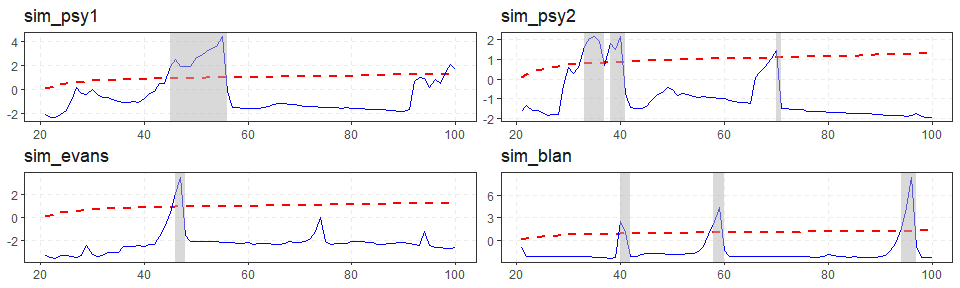However, almost all aspects of the plot can be easily changed.

### Change color and theme

The custom color for autoplot are “blue and”red", however the user can easily override this option with ggplot2::scale_color_manual.

autoplot(estimation, crit_values) +
scale_color_manual(values = c("grey","black")) +
theme_classic()
#> Warning: Removed 1 rows containing missing values (geom_rect).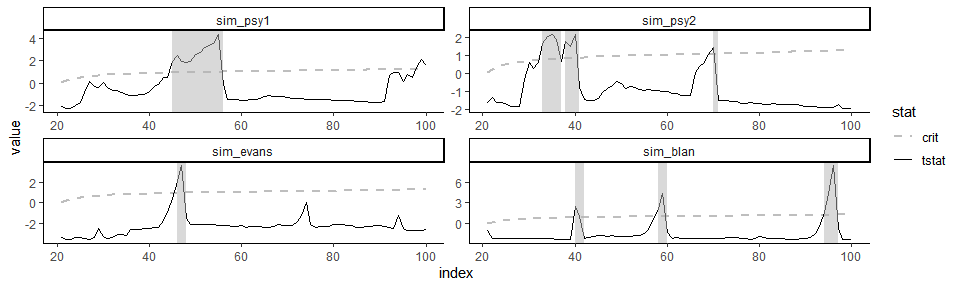### Changed the shaded region with shade_opt

shade_opt allows the user to manipulate the geom_rect() layer of the ggplot, using the shade function. Alternatively, it can be omitted if it set to NULL.

autoplot(estimation, crit_values, shade_opt = shade(fill = "pink", opacity = 0.3))
#> Warning: Removed 1 rows containing missing values (geom_rect).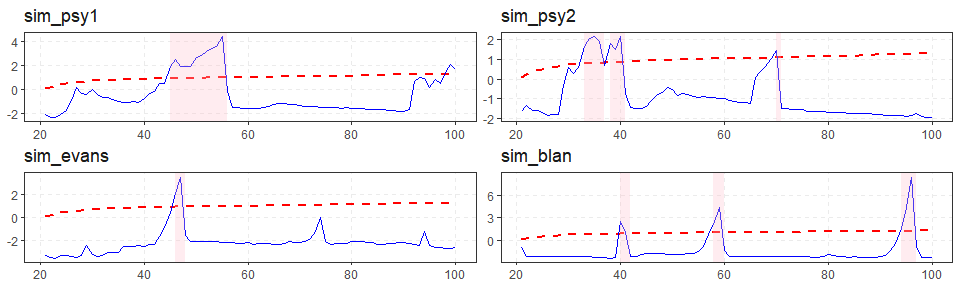## Custom plotting

Custom plotting is also very easy with the augment_join(), that merge the output of the estimation and and critical values in a ggplot2-friendly way.

joined <- augment_join(estimation, crit_values)
joined
#> # A tibble: 1,920 x 7
#>      key index id       name   tstat sig    crit
#>    <int> <dbl> <fct>    <fct>  <dbl> <fct> <dbl>
#>  1    21    21 sim_psy1 badf  -2.08  90    -0.44
#>  2    22    22 sim_psy1 badf  -2.31  90    -0.44
#>  3    23    23 sim_psy1 badf  -2.39  90    -0.44
#>  4    24    24 sim_psy1 badf  -2.26  90    -0.44
#>  5    25    25 sim_psy1 badf  -2.08  90    -0.44
#>  6    26    26 sim_psy1 badf  -1.79  90    -0.44
#>  7    27    27 sim_psy1 badf  -1.00  90    -0.44
#>  8    28    28 sim_psy1 badf  -1.34  90    -0.44
#>  9    29    29 sim_psy1 badf  -1.28  90    -0.44
#> 10    30    30 sim_psy1 badf  -0.800 90    -0.44
#> # ... with 1,910 more rows

The output of augment_join returns data in tidy format and offers full flexibility to the user. After this point plotting becomes extremely trivial.

joined %>%
ggplot(aes(x = index)) +
geom_line(aes(y = tstat)) +
geom_line(aes(y = crit)) +
facet_grid(sig + name ~  id  , scales = "free_y")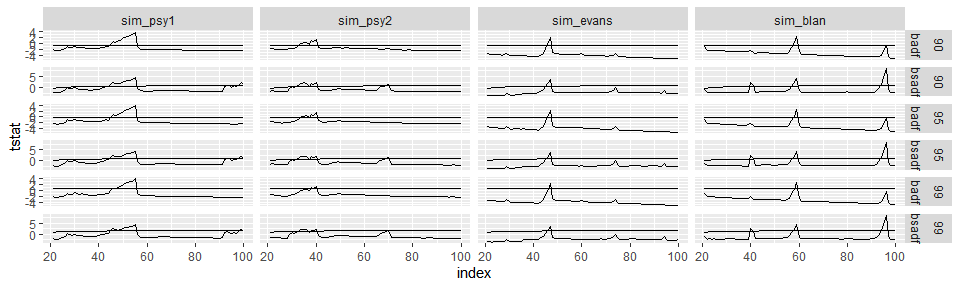We also offer two functions scale_exuber_manual and theme_exuber that offer some extra functionality.

joined %>%
pivot_longer(cols = c("tstat", "crit"), names_to = "nms") %>%
ggplot(aes(x = index, y = value, col = nms)) +
geom_line() +
facet_grid(sig + name ~  id  , scales = "free_y") +
scale_exuber_manual() +
theme_exuber()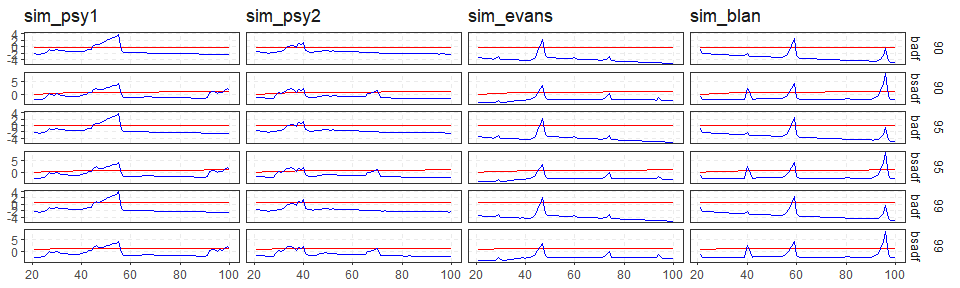## Distribution

In addition to critical values, we can also calculate the empirical distribution by utilizing the family of *_distr functions. For example if we can simulate the distribution of the supADF tests with Monte Carlo method.

distr <- radf_mc_distr(n = 300)
autoplot(distr)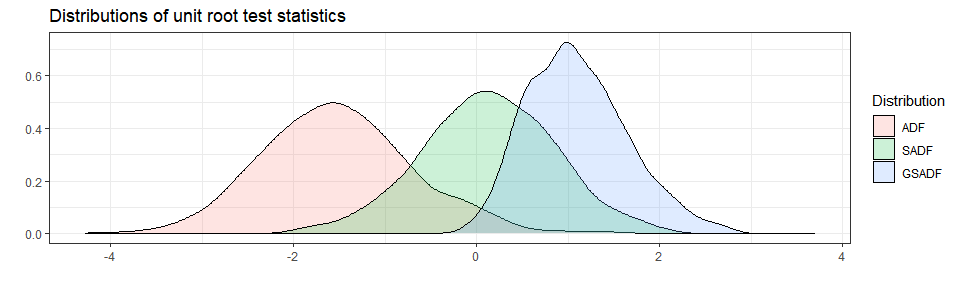### Empirical distribution

This part is made just for fun.

library(tidyr)
distr %>%
tidy() %>%
rename_all(~ stringr::str_to_upper(.)) %>%
gather(Statistic, value, factor_key = TRUE) %>%
ggplot(aes(value, color = Statistic)) +
stat_ecdf() +
ggtitle("Empirical Cumulative Distribution") +
geom_hline(yintercept = 0.95, linetype = "dashed") + theme_bw()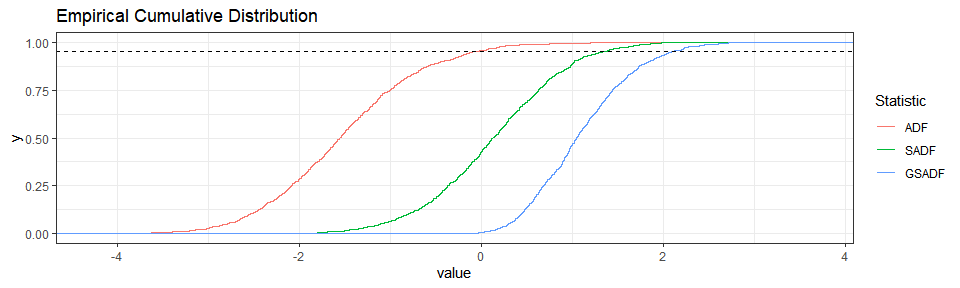## Old Functionality

To return to the old functionality there are several ways.

library(gridExtra)

# To choose only positive series (i.e. statistically significant for 5%)
positive_series <- diagnostics(estimation, crit_values)\$positive

# Through a loop on positive series
plot_list1 <- list()
for (as in positive_series) {
plot_list1[[as]] <- autoplot(estimation, crit_values, select_series = as)
}

# Alternatively  with lapply
plot_list2 <- lapply(positive_series, function(x) autoplot(estimation, crit_values, select_series = x))
names(plot_list2) <- positive_series

do.call(gridExtra::grid.arrange, plot_list1)
#> Warning: Removed 1 rows containing missing values (geom_rect).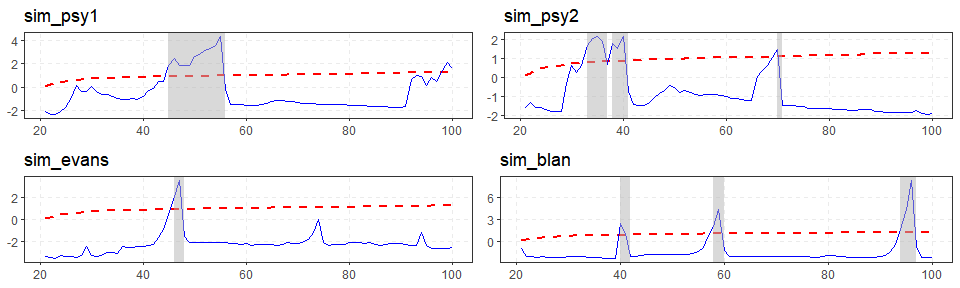With the old functionality you had to make changes one at a time

plot_list1[] <- plot_list1[] + theme_classic()

and then reconstruct the plot with grid.arrange or some other function that arranges all plots into a single grob.

Enjoy Plotting with exuber !!!